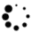# Use a simple vapor equation for sizing two-phase pressure relief valves

Pressure relief valves (PRVs) protect process equipment from the hazards of excessive over-pressure.

Pressure relief valves (PRVs) protect process equipment from the hazards of excessive over-pressure. A simple liquid equation is widely used as a standard method for sizing incompressible fluids. A simple vapor equation is also widely used when sizing for ideal vapors. For non-ideal vapors, an isentropic expansion coefficient typically evaluated at the relief conditions is used. Alternatively, API 520 Part 1 suggests averaging the isentropic expansion coefficients calculated at the upstream pressure and the pressure in the throat of the valve nozzle.1 Using the average isentropic expansion coefficient with a simple vapor equation can result in undersized PRVs due to the assumption of an ideal vapor in the simple vapor equation.

This article proposes an alternative solution for a constant isentropic expansion coefficient. For two-phases, the Leung Omega method—often referred to as a two-point method—is widely used. In addition, the homogeneous direct integration (HDI) method requires significant computational efforts but is considered the most rigorous and accurate method.

No standardized PRV specification sheet is in use for non-ideal vapors and two-phases because no simple unified methodology exists for compressible fluids (non-ideal vapors and two-phases). For two-phases, the mass flux and the basis for the calculations are specified in accordance with the API 520 Part 1, Annex D.1 However, no guidelines are available for non-ideal vapors. This article introduces a new method for sizing any compressible fluid PRV using a simple vapor equation. The proposed approach generates rigorous results validated against the existing rigorous HDI method. This method is applicable to vapors and two-phases, including slightly subcooled liquids if the three-point data is available from isentropic flash calculations. In addition, it significantly reduces the computational efforts necessary for the numerical integration method. The new method using a simple vapor equation will improve the communication between the valve manufacturer and the relief system engineer for non-ideal vapors and two-phases, including slightly subcooled liquids.

## A simple vapor equation

For vapors, the critical flow through a PRV is represented by Eq. 1, which assumes the vapor behaves ideally when a compressible vapor expands across a PRV nozzle. The simple vapor equation provides satisfactory sizing results over a wide range of applications. However, to ensure the appropriate sizing results, the vapor compressibility factor should be no less than approximately 0.8 or greater than approximately 1.1.1 Recently, API 520 Part 1 noted that the validity of Eq. 1 may diminish when the reduced volume of the fluid is less than 2 at the valve inlet pressure: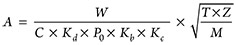(1)

where,

A = the required discharge area of the device, in.2
W = the required flow through the device, lb/hr
C = a function of the ratio of the ideal gas-specific heats at the inlet relieving temperature
Kd = the coefficient of discharge
P0 = the inlet relieving pressure, psia
Kb = the capacity correction factor due to backpressure
Kc = the combination correction factor for installations with a rupture disk upstream of PRV
T = the relieving temperature of the inlet vapor, °R
Z = the compressibility factor at inlet relieving conditions
M = the molecular weight of the vapor at inlet relieving conditions.

For real gases, API 520 Part 1 recommends using an isentropic expansion coefficient evaluated at the relief conditions. Note: A real gas-specific heat ratio does not provide a good representation of the isentropic expansion coefficient.1 Alternatively, an average isentropic expansion coefficient between the upstream pressure and the pressure in the throat of the nozzle is recommended.1 However, the isentropic expansion coefficient is not used in the API 520 PRV specification sheets.

## Numerical integration method

API 520 Part 1 contains the most rigorous method for calculating the mass flux for PRVs. The method applicable to all phases is known as the HDI method, evaluated numerically by direct summation over small pressure intervals using an appropriate technique (e.g., Simpson’s method).2 The HDI method can be used for any homogeneous fluid provided fluid property data at constant entropy is available. The critical mass flux can be estimated by integrating (as shown in Eq. 2) until the mass flux reaches a maximum mass flux. If the fluid is subcritical, the subcritical mass flux can be estimated by integrating until the actual backpressure on the nozzle is reached, as shown in Eq. 3: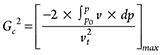(2)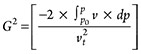(3)

where,

G = the mass flux through the nozzle, lb/sec-ft2
P = the pressure of the fluid, psia
v = the specific volume of the fluid, ft3/lb
t = the throat of the nozzle.

The calculation precision associated with the HDI method is determined by the size of the pressure intervals, with smaller pressure intervals providing better results. Some cases may require many data sets for acceptable sizing results. The HDI method requires intensive computational efforts; however, the numerical integral result can also be obtained accurately and quickly if evaluated analytically with pressure-specific volume (P-v) models or employed P-v models.

## Reduced P-v models

The equation of state (EOS) typically involves four variables. Simpson presented a total of six reduced P-v models involving only two variables.2 The reduced P-v model is obtained by a few constant entropy flash calculations, for which the specific volume reflects changes in the compressibility factor, pressure and temperature. The reduced P-v model based on the homogeneous equilibrium model (HEM) well represents the pressure expansion processes in the PRV.

This article evaluates two out of the six P-v models and a conventional model in a simple vapor equation to select the best P-v model for isentropic expansion flow. Eq. 4 is good for ideal vapors, but not suitable for flashing liquids. Eq. 5, the Omega method, is good for flashing liquids, but not so suitable for vapors. The two-point Omega method is most widely used for sizing two-phases. Eq. 6 is a combination of Eqs. 4 and 5, so it should be suitable for both vapors and two-phases. Simpson noted that Eq. 6 is the only reduced P-v model that gave consistently outstanding fits of the data.(4), (5), (6)

where,

n,ω,α,β = the P-v model constants.

For subcooled liquids, the initial pressure (P0) shall be at the saturated pressure, ignoring subcooled conditions. Eq. 5, a two-point method, uses two data sets at initial pressure and typically 90% of the initial pressure. Eq. 6, a three-point method, uses three data sets at initial pressure, a middle pressure and 50% of the initial pressure (not greater than the critical pressure).

## Upgrading the numerical integration method

A new approach to make the rigorous HDI method more convenient is discussed here. To reduce significant computational efforts, Kim, et al. proposed Eqs. 7–9, which are based on Eq. 6.3 A compressible fluid reaches a condition of maximum flow when the velocity at the nozzle reaches the speed of sound. The isentropic maximum mass flux for Eq. 2 is given by Eq. 7. Eqs. 7 and 8 are in an identical derivative form.

An identical and simple equation form for both critical mass flux and subcritical mass flux is preferred to simplify the calculation method. This allows for an analytical solution for mass flux calculations to make the numerical integration easier. Eqs. 2 and 3 are in an identical integral form rather than derivative. The integral form does not allow the analytical solution for mass flux calculations. Eq. 9 is derived from Eqs. 3 and 8, and has been upgraded here to handle slightly subcooled liquids. Eq. 9 simplifies the numerical integration and provides rigorous results. Both Eqs. 7 and 8 provide the results of P-v equation-based integration. This new method is applicable to compressible fluids (vapors and two-phases, including slightly subcooled liquids):(7), (8), (9)

where,

ec–max = the critical pressure (Pc), maximum equivalent pressure
ec = the equivalent critical pressure at the calculated mass flux
sc = the subcooled liquid.

Eq. 10 has been developed to determine if a subcooled liquid is compressible or incompressible. Eq. 10 is similar to the API 520 Part 1 Equation (C.32), but easier to follow. If the subcooled pressure is greater than the calculated Pecmax, the fluid is incompressible; the fluid is not considered a slightly subcooled liquid because there is no change in density during the expansion process. In this case, the fluid should be sized using a liquid equation and the fluid chokes at the saturated pressure. If the subcooled pressure is not greater than the calculated Pecmax, the fluid is compressible. In this case, the fluid should be sized as a two-phase fluid: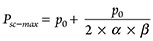(10)

For subcritical mass flux, use Eqs. 8 and 9. The Pec in Eq. 8 is calculated using Eq. 9 at P (typically at backpressure) without iteration. For critical mass flux, use Eqs. 7 and 9. The Pecmax in Eq. 7 is also calculated using Eq. 9 at P (initially at backpressure) with iteration until Pec is equal to P. The calculated Pec replaces P in the next trial. The maximum Pec value (Pec–max) is obtained when Pec is equal to P.

Critical pressure can also be obtained from the following approximations (Eqs. 11 and 12) developed based on the Omega method.(11), (12)

All values of nozzle pressure and Pec, along with mass flux, are plotted in FIG. 1 to tangibly illustrate the relations among nozzle pressure, Pec, Pc and mass flux for API 520 Part 1, Example B.3.3. The green line shows the subcritical mass flux calculations for a known backpressure (at P, greater than the critical pressure). In subcritical flow, there is no iteration. Because Eq.9 provides a result of equivalent critical pressure at the integrated mass flux from initial pressure to the backpressure, the calculated Pec is directly used for the mass flux calculation. The calculated Pec value shall be less than the known backpressure (P).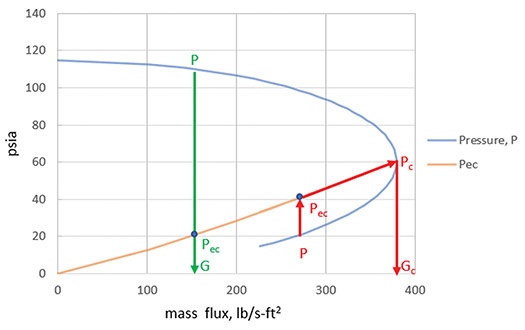FIG. 1. Plot of nozzle pressure, Pec and mass flux.

The red line shows the critical mass flux calculations for a known backpressure (at initial P, the backpressure is not greater than the critical pressure). In critical flow, the calculations are repeated until the calculated Pec is equal to P. The mass flux and Pec are maximized at the critical pressure (Pc). In the critical flow region, the maximum mass flux is taken at Pc because a further decrease in nozzle pressure does not affect the mass flux. The calculated Pec also decreases as the P is further decreased below the critical pressure.

The HDI method uses only one blue graph. The upgraded integration method uses two graphs (blue graph and gold graph). The two graphs intersect at Pc. Using the two graphs significantly improves obtaining the critical pressure point quickly and accurately. Eq. 9 is used to calculate both the Pec for subcritical mass flux and the Pecmax (Pc) for critical mass flux. The upgraded integration method quickly determines if the flow is critical or subcritical. However, the HDI method should complement the Eq. 2 calculations to determine if the flow is critical or subcritical.

## Isentropic expansion coefficient

Estimating the isentropic expansion coefficient during the expansion process is not simple because it is a function of pressure, volume and the ratio of real gas-specific heats, as shown in Eq. 13. It also varies throughout the expansion process, during which condensation may occur. The isentropic expansion coefficient for non-ideal vapors at the relief conditions is often used because it is readily available. A constant isentropic expansion coefficient can be easily back-calculated using Eq. 14 if the C value is known. Eq. 14 can be expressed in terms of two-parameters in a P-v model, critical pressure and initial relief pressure, as shown in Eq. 15. The C value can be calculated by other methods if the mass flux result is validated. Eq. 15 is recommended as the calculation method of choice as a result of the reduced P-v model evaluation.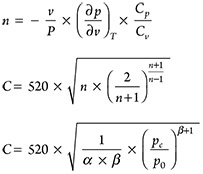(13), (14), (15)

Kim proposed Eqs. 15–17 at the API SCPRC Meeting.4 Eq. 15 is derived from Eqs. 1 and 7.

The polynomial Eqs. 16 and 17 are used to estimate the constant isentropic expansion coefficient for two different C ranges. The C value is a result of the most rigorous calculation method. This may be an outstanding solution for constant isentropic expansion coefficients. This approach allows a simple vapor equation to be extended to any compressible fluid, including slightly subcooled liquids because Eq. 1 is based on Eq. 4. Therefore, a simple vapor equation can be applied to any compressible fluid, not only to ideal vapors.

However, n should be a constant isentropic expansion coefficient obtained from Eq. 14 to be compatible with a simple vapor equation. The constant isentropic expansion coefficient reflects the assumption of ideal vapor relationship in Eq. 14 since it is simplified for ideal vapors with the ratio of the ideal gas-specific heats; therefore, the constant isentropic expansion coefficient is different from the average isentropic expansion coefficient. For a non-ideal vapor, an average isentropic expansion coefficient can result in undersized PRVs. For an ideal vapor, an ideal gas-specific heat ratio at the relief inlet conditions can be used as a constant isentropic expansion coefficient.

For 12 < C ≤ 160:

n = 2.5343E-11 × C4–8.3347E-10 × C3 + 7.4632E-6 × C2–2.433E-6 × C + 3.3047E-5                                                                (16)

For C > 160:

n = 3.8459E-13 × C5 – 3.7986E-10 × C4 + 1.7953E-7 × C3–3.3542E-5 × C2 + 4.6767E-3 × C – 2.1238E-1                                   (17)

## A new method for sizing compressible fluids

Sizing methods for significantly non-ideal vapors and two-phases—including slightly subcooled liquids—have not yet been standardized. However, many effective new methods have been developed over the last few decades. A new method for sizing compressible fluids using a simple vapor equation is proposed here to be a standard sizing method. All equations required for the new method are summarized here based on the standard steps in a work procedure (Eqs. 1, 6, 9, 10, 15, 16 and 17 ):(1)(6)(9)(10)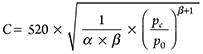(15)

For 12 < C ≤ 160:

n = 2.5343E-11 × C4–8.3347E-10 × C3 + 7.4632E-6 × C2–2.433E-6 × C + 3.3047E-5                                                             (16)

For C > 160:

n = 3.8459E-13 × C5 – 3.7986E-10 × C4 + 1.7953E-7 × C3–3.3542E-5 × C2 + 4.6767E-3 × C – 2.1238E-1                               (17)

## Detailed standard steps in a work procedure

The following procedure can be used for sizing compressible fluids (including slightly subcooled liquids) using a simple vapor equation:

1. Calculate the two parameters, α and β, in Eq. 6 using three pressure-specific volume data:
• Two flash calculations should be carried out at constant entropy
• P0 is at the PRV inlet
• P0 is at the saturated pressure for slightly subcooled liquids; do not include the subcooled region
• P2 is at the backpressure or 50% of P0 (not greater than the critical pressure)
• Higher P2 would improve the calculation accuracy
• P1 is the average pressure of P0 and P2
• Use a goal seek feature or a simple macro program.
1. Calculate the C value in Eq. 15:
• For subcooled liquids, determine if the fluid is compressible or incompressible using Eq. 10; do not use this method for incompressible fluids
• Psc = P0 if the fluid is not subcooled
• Specify the Psc if the fluid is slightly subcooled (PscPsc–max)
• Calculate the Pc (Pec–max) iteratively using Eq. 9 until the Pec is equal to P; alternatively, Eq. 11 can be used to avoid iteration
• For a subcritical flow with conventional valves, calculate the Pec without iteration; use the Pec instead of Pc (Pec–max) and use Kb = 1, or calculate the Pc (Pec–max) using Eq. 9 until the Pec is equal to P and use Kb = Cat Pec/Cat Pc
• For a subcritical flow with balanced valves, use the Pc (Pec–max), and apply the backpressure correction factor (Kb) supplied by valve manufacturers
• Use a goal seek feature or a simple macro program.
1. Calculate the n using Eqs. 16 and 17:
• n (constant isentropic expansion coefficient) is specified on the PRV specification sheet.
1. As usual , size PRVs using Eq. 1:
• Eq. 1 can size vapors and two-phases, including slightly subcooled liquids.

## Validation of new method

This section is included to validate the proposed method by comparing it with the existing HDI and Omega methods using readily available example problems from API 520 Part 1—API 520 Part 1 does not cite an example for slightly subcooled liquids. Case 1 is from Simpson’s Example 1.2 TABLE 1 shows comparisons with different sizing method results for the following four cases.

Case 1 : Simpson’s Example 1
Fluid: Subcooled water at 66 psia and 298°F (148°C), saturated at 65 psi
HDI: Used 12-point data at 0.2 psi constant pressure intervals
Omega: Used two-point data at 65 psia (0.017433 ft3/lb) and 58.5 psia (0.074177 ft3/lb)
New: Used three-point data at 65 psia (0.017433 ft3/lb), 48 psia (0.205211 ft3/lb), and 32 psia (0.612741 ft3/lb)

Case 2: API 520 Part 1, Example Problem C.2.1.2
Fluid: Two-phase containing a significant amount of hydrogen at 2,168.5 psia and 80.3°F (27°C)
HDI: Used 14-point data at 86.7 psi constant pressure intervals
Omega: Used two-point data at 2,168.5 psia (5.18 lb/ft3) and 1,908.3 psia (4.77 lb/ft3)
New: Used three-point data at 2,168.5 psia (5.18 lb/ft3), 1,648.1 psia (4.28 lb/ft3) and 1,040.9 psia (2.96 lb/ft3

Case 3: API 520 Part 1, Example Problem B.1.3
Fluid: Supercritical ethylene at 797.7 psia and 80.33°F (27°C)
HDI: Used 44-point data at 7.8 psi constant pressure intervals
Omega: Used two-point data at 797.7 psia (0.152 ft3/lb) and 719.4 psia (0.165 ft3/lb)
New: Used three-point data at 797.7 psia (0.152 ft3/lb), 625.4 psia (0.185 ft3/lb) and 445.3 psia (0.248 ft3/lb)

Case 4: API 520 Part 1, Example Problem B.3.3
Fluid: Low-pressure air at 114.7 psia and 80.33°F (27°C)
HDI: Used 60-point data at 1 psi constant pressure intervals
Omega: Used two-point data at 114.7 psia (1.741 ft3/lb) and 103.7 psia (1.870 ft3/lb)
New: Used three-point data at 114.7 psia (1.741 ft3/lb), 85.7 psia (2.141 ft3/lb) and 55.7 psia (2.907 ft3/lb)

A simple vapor equation satisfactorily sizes PRVs for all four cases. Red color values in TABLE 1 represent the deviation in mass flux of > 2% and the deviation in critical pressure more than the constant pressure interval from the HDI method. The new method is in excellent agreement with the HDI method for all four cases. Blue color values in TABLE 1 represent calculated C values and constant isentropic expansion coefficients for all four cases.

For Case 1 (slightly subcooled water), a commercial process simulator using the ASME steam property package provides the average isentropic expansion coefficient of 0.0729. The ASME steam specific volume data is in good agreement with the Case 1 data. The new method predicts the constant isentropic expansion coefficient of 0.0671. The average isentropic expansion coefficient with a simple vapor equation results in undersized PRVs. This fluid is compressible because Psc, 66 psia ≤ calculated Psc–max, 66.13 psia. The C value and constant isentropic expansion coefficient are much less than the other cases. The new method predicts better critical pressure than the HDI method because Simpson used specific volume data from the same P-v model and numerically integrated at a constant pressure interval of 0.2 psi.

For Case 2 (two-phase containing hydrogen), there is no composition information available to evaluate the constant isentropic expansion coefficient.

For Case 3 (supercritical ethylene), a commercial process simulator using the SRK (Soave-Redlich-Kwong) property package provides the average isentropic expansion coefficient of 1.2424. The SRK specific volume data agrees well with the Case 3 data. The new method predicts a constant isentropic expansion coefficient of 1.1863. The average isentropic expansion coefficient with a simple vapor equation results in slightly undersized PRVs.

For Case 4 (low-pressure air), a commercial process simulator using the SRK (Soave-Redlich-Kwong) property package gives the average isentropic expansion coefficient of 1.4203. The SRK specific volume data is in good agreement with the Case 4 data. The new method predicts the constant isentropic expansion coefficient of 1.4091. This is an acceptable difference in two isentropic expansion coefficients for air. This confirms that Eq. 14 is acceptable for ideal vapors or relatively constant isentropic expansion coefficients.

## Takeaways

The above proposed method for sizing compressible fluid PRVs using a simple vapor equation is an outstanding approach. The new method is based on the classical homogeneous equilibrium model chosen by API 520 Part 1 and AIChE/DIERS for emergency pressure relief system designs. Most pressure relief system engineers are familiar with the simple vapor equation.

For non-ideal vapors, an average isentropic expansion coefficient does not give the corresponding correct mass flux because Eq. 14 assumes ideal vapor. Generally, using the average isentropic expansion coefficient with a simple vapor equation can result in undersized PRVs. The new method provides the most practical solution for a constant isentropic expansion coefficient that is compatible with a simple vapor equation. For ideal vapors, an ideal gas-specific heat ratio at the relief inlet conditions can be used as a constant isentropic expansion coefficient. The proposed method allows a simple vapor equation to size compressible fluids (vapors and two-phases including slightly subcooled liquids) if the three-point data is available from isentropic flash calculations. This approach produces rigorous results validated against the existing rigorous HDI method, while significantly reducing computational efforts necessary for the numerical integration method.

Presently, there is no standardized PRV specification sheet for non-ideal vapors and two-phases. The proposed method using a simple vapor equation will improve the communication between the valve manufacturer and the relief system designer for non-ideal vapors and two-phases, including slightly subcooled liquids. The proposed method using a simple vapor equation is recommended as the standard sizing methodology for compressible fluids, including slightly subcooled liquids. HP

LITERATURE CITED

1. American Petroleum Institute (API) Standard 520 Part 1, “Sizing, selection and installation of pressure-relieving devices: Part I—Sizing and selection,” 10th Ed., October 2020.
2. Simpson, L. L., “Navigating the two-phase maze,” International Symposium on runaway reactions and pressure relief design, August 2–4 1995.
3. Kim, J. S., et al., “Sizing PRVs,” Chemical Engineering, February 2013.
4. Kim, J. S., “P-v based integration and speed of sound,” API Spring SCPRS Meeting, May 2021.
5. Melhem, G. A., “Reduced analytical models for flow hydraulics,” AIChE/DIERS Fall Meeting, Houston, Texas, October 2018.

## From the Archive